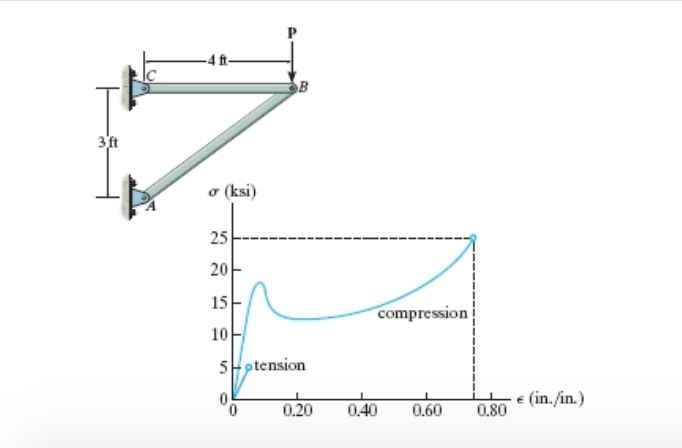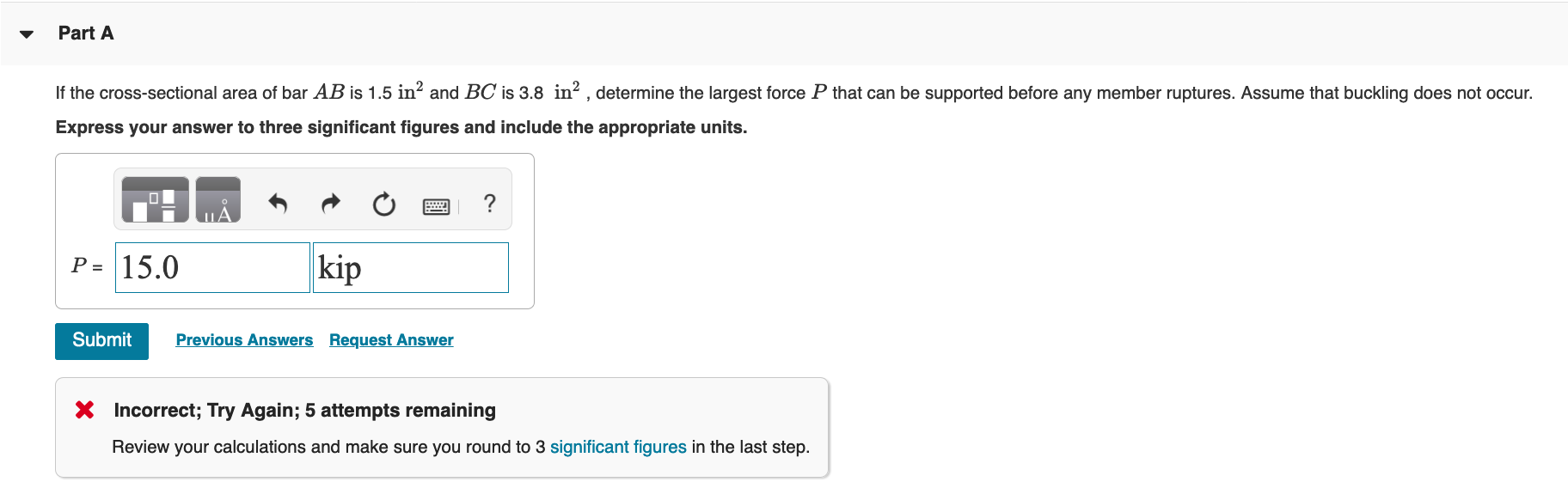# (Solved): The Two Bars Are Made Of Polystyrene, Which Has The Stress-strain Diagram Shown In The Figure Below....The two bars are made of polystyrene, which has the stress-strain diagram shown in the figure below. (Figure 1) o (ksi) compression 5ptension "O 0.20 0.40 0.60 0.80 (in./in.) Part A If the cross-sectional area of bar AB is 1.5 inand BC is 3.8 inÂ² , determine the largest force P that can be supported before any member ruptures. Assume that buckling does not occur. Express your answer to three significant figures and include the appropriate units. P= 15.0 kip Submit Previous Answers Request Answer * Incorrect; Try Again; 5 attempts remaining Review your calculations and make sure you round to 3 significant figures in the last step.

We have an Answer from Expert